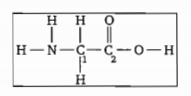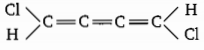What are the hybridisation of the carbon atoms labelled ${C}_{1}$ and ${C}_{2}$ respectively in glycein?${C}_{1}$             ${C}_{2}$

(a) $s{p}^{2}$         (a) $s{p}^{2}$

(b) $s{p}^{2}$         (b) $s{p}^{3}$

(c) $s{p}^{3}$          (c) $s{p}^{2}$

(d) $sp$          (d) s${p}^{3}$

Concept Questions :-

Hybridisation
High Yielding Test Series + Question Bank - NEET 2020

Difficulty Level:

Choose the correct option for the following molecule :(a) non planar

(b) $\mu \ne 0$

(c) both (a) and (b)

(d) $\mu =0$

Concept Questions :-

Polarity
High Yielding Test Series + Question Bank - NEET 2020

Difficulty Level:

Select the correct statement:

(a)$HS{O}_{5}^{-}$ ion has one $\mathrm{S}—\mathrm{O}—\mathrm{H}$ linkage

(b) Number of $\mathrm{B}—\mathrm{O}—\mathrm{B}$ linkages in Borax is equal to number of $\mathrm{P}—\mathrm{O}—\mathrm{P}$ linkages in ${\mathrm{P}}_{4}{\mathrm{O}}_{10}$

(c) Hybridization of both sulphur on $\left({\mathrm{H}}_{2}{\mathrm{S}}_{2}{\mathrm{O}}_{5}\right)$ (pyrosulphurous acid) is name but oxidization state of both sulphur are different

(d) Tetra -Polyphosphoric has four $\mathrm{P}—\mathrm{O}—\mathrm{P}$ and no $\mathrm{P}—\mathrm{P}$ linkage

Concept Questions :-

Hybridisation
High Yielding Test Series + Question Bank - NEET 2020

Difficulty Level:

The H-O-H bond angles in ${H}_{3}{O}^{+}$ are approximately ${107}^{°}$. The orbitals used by oxygen in these bond are best describe as:

(a) $p-orbitals$

(b) sp-hybrid orbitals

(c) hybrid orbital

(d) $s{p}^{3}$. hybrid orbital

Concept Questions :-

Hybridisation
High Yielding Test Series + Question Bank - NEET 2020

Difficulty Level:

Which of the following molecular species is not linear?

(a) $\left(\mathrm{CN}{\right)}_{2}$                                                       (b) ${\mathrm{OCN}}^{-}$

(c) ${\mathrm{XeF}}_{2}$                                                         (d) ${\mathrm{S}}_{3}^{2-}$

Concept Questions :-

Hybridisation
High Yielding Test Series + Question Bank - NEET 2020

Difficulty Level:

Which of the following statement is correct about ${{I}_{3}}^{+}$and ${{I}_{3}}^{-}$ molecure ions?

(a) Number of lone pairs at central atoms are samein both molecure ions

(b) Hybridization of central atoms in both ions are same

(c) both are polar spacies

(d) both are planar spacies

Concept Questions :-

Hybridisation
High Yielding Test Series + Question Bank - NEET 2020

Difficulty Level:

The compound that has the highest ionic character assoicated with the X-Cl bond is:

(a)$PC{l}_{5}$

(b) $BC{l}_{3}$

(c) $CC{l}_{4}$

(d)$SiC{l}_{4}$

Concept Questions :-

Polarity
High Yielding Test Series + Question Bank - NEET 2020

Difficulty Level:

In which of the folllowing transformations, the bond order has increased and the magnetic behaviour has changed?

(a) ${\mathrm{C}}_{2}^{+}\to {\mathrm{C}}_{2}$                      (b) ${\mathrm{NO}}^{+}\to \mathrm{NO}$

(c) ${\mathrm{O}}_{2}\to {\mathrm{O}}_{2}^{+}$                       (d) ${\mathrm{N}}_{2}\to {\mathrm{N}}_{2}^{+}$

Concept Questions :-

M.O.T
High Yielding Test Series + Question Bank - NEET 2020

Difficulty Level:

The bond having the highest bond energy is :

(a) C=C

(b) C=S

(c) C=O

(d) P=N

High Yielding Test Series + Question Bank - NEET 2020

Difficulty Level:

H.O.M.O. (Highest Occupied Molecular Orbital) of CO molecular is :

(a) Non-bonding M.O. with slight antibonding character

(b) Non-bonding M.O. with slight bonding character

(c) Pure non-bonding M.O.

(d) None of the above

Concept Questions :-

V.S.E.P.R